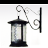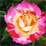# 'IF' Python Script Tool

1599
16
09-08-2010 09:37 AMNew Contributor
I need to mimic an Excel formula as an ArcGIS script tool (I will run many times on similar fields).  It includes the following:
-1 feature class:   'gp.GetParameterAsText(0)'
-3 input fields:   (Z, AF, T)   'gp.GetParameterAsText(1), (2), & (3)'
-1 output field:   'gp.GetParameterAsText(4)'

In Excel, the formula looks like this:
=IF(AND(Z>0,AF>0),SUM(T+Z+AF),IF(AND(Z>0,AF<0),SUM(T+Z),IF(AND(Z<0,AF>0),SUM(T+AF),T)))

In plain words, it is saying:
If the first condition is met, sum these 3 fields together and populate output field
If the second condition is met, sum these 2 fields together and populate output field
If the third condition is met, sum these 2 fields together and populate output field
If none are met, populate output field with field 'T' value.

I am somewhat familiar with Python, but I am struggling with this one.  Any help is appreciated.  Thank you.
Tags (3)
16 RepliesbyMVP Esteemed Contributor
IF(AND(Z>0,AF>0),SUM(T+Z+AF),IF(AND(Z>0,AF<0),SUM (T+Z),IF(AND(Z<0,AF>0),SUM(T+AF),T)))

given Z, AF and T as variables, written explicitly
```....stuff before
if (Z > 0) and (AF > 0):
x = sum( [T, Z, AF] )    #or x = T + Z + AF
elif (Z > 0) and (AF < 0):
x = sum( [T, Z ] )         #or x = T + Z
elif (Z < 0) and (AF > 0):
x = sum( [T, AF] )        #or x = T + AF
else:
x = T
....
stuff after
```

you sum lists, ie.
>>> a = [1,2,3]
>>> sum(a)
6
>>>Occasional Contributor III
This should be a piece of cake in Python:

2. Get an UpdateCursor from the feature class

3. For each row in the cursor, do the same logic (psuedo-code):

4. ```if [condition 1]: # first condition to check
[set field value]
elif [condition n]: # you'll have several instances of this for every other condition
[set field value]
else: # no other conditions matched
[set field value]
[update the row]
[move to the next row]
```

5. Clean up references to row and cursorNew Contributor
I think I am close (with your help of course); but what do you think is wrong with this?  It runs without completing, on a relativley small sample.

```FC = gp.GetParameterAsText(0)
Z = gp.GetParameterAsText(1)
AF = gp.GetParameterAsText(2)
T = gp.GetParameterAsText(3)
Outfield = gp.GetParameterAsText(4)

cur = gp.UpdateCursor(FC)
row = cur.Next()

while row:
if (Z > 0) and (AF > 0):
Outfield = T + Z + AF    #or = sum( [T, Z, AF] )
elif (Z > 0) and (AF < 0):
Outfield = T + Z         #or = sum( [T, Z ] )
elif (Z < 0) and (AF > 0):
Outfield = T + AF        #or = sum( [T, AF] )
else:
Outfield = T
del cur```byMVP Esteemed Contributor
change the
row = cur.Next() to row = cur.next() if using 10
```while row:
if (Z > 0) and (AF > 0):
Outfield = T + Z + AF    #or = sum( [T, Z, AF] )
elif (Z > 0) and (AF < 0):
Outfield = T + Z         #or = sum( [T, Z ] )
elif (Z < 0) and (AF > 0):
Outfield = T + AF        #or = sum( [T, AF] )
else:
Outfield = T
row = cur.next()   # <-------------missing the move to the next row
```Occasional Contributor III
I think I am close (with your help of course); but what do you think is wrong with this?  It runs without completing, on a relativley small sample.

```FC = gp.GetParameterAsText(0)
Z = gp.GetParameterAsText(1)
AF = gp.GetParameterAsText(2)
T = gp.GetParameterAsText(3)
Outfield = gp.GetParameterAsText(4)

cur = gp.UpdateCursor(FC)
row = cur.Next()

while row:
if (Z > 0) and (AF > 0):
Outfield = T + Z + AF    #or = sum( [T, Z, AF] )
elif (Z > 0) and (AF < 0):
Outfield = T + Z         #or = sum( [T, Z ] )
elif (Z < 0) and (AF > 0):
Outfield = T + AF        #or = sum( [T, AF] )
else:
Outfield = T
del cur```

All you're currently doing is setting the value of the Outfield variable. You need to be setting the value of the field itself, and then updating the row. Since your field name is a variable, use the SetValue command to set the field's value, and use the UpdateRow command to actually commit the change to the database. And like Dan mentioned, remember to move to the next row before the next iteration.byMVP Esteemed ContributorNew Contributor
Thank you for the assistance.  With the modifications (below), I now get an error when running the script tool.  Am I close here?  Any help is appreciated.  Thanks.
```FC = gp.GetParameterAsText(0)
Z = gp.GetParameterAsText(1)
AF = gp.GetParameterAsText(2)
T = gp.GetParameterAsText(3)
Outfield = gp.GetParameterAsText(4)

cur = gp.UpdateCursor(FC)
row = cur.Next()

while row:
if (Z > 0) and (AF > 0):
row.SetValue(Outfield, T + Z + AF)  #or = sum( [T, Z, AF] )
cur.UpdateRow(row)
elif (Z > 0) and (AF < 0):
row.SetValue(Outfield, T + Z)         #or = sum( [T, Z ] )
cur.UpdateRow(row)
elif (Z < 0) and (AF > 0):
row.SetValue(Outfield, T + AF)        #or = sum( [T, AF] )
cur.UpdateRow(row)
else:
row.SetValue(Outfield, T)
cur.UpdateRow(row)
row = cur.Next()
del cur```byMVP Esteemed Contributor
With respect...and sensing a bit of anticipation on your part...mind readers we are not...what is the error message?
version of ArcMap?
version 9.x operates differently than 10 for example UpdateRow in 9.3 is updateRow in version 10 as is the setValue methodOccasional Contributor III
Try creating a variable to store the value you're calculating in the SetValue command instead of doing the calculation in-line. I don't know why, but this appears to be a problem with some ESRI arcgisscripting commands.

for example:
```      if (Z > 0) and (AF > 0):
val = T + Z + AF #or = sum( [T, Z, AF] )
row.SetValue(Outfield, val)
cur.UpdateRow(row)
```

Also, I don't know what T, Z and AF are, but since we're only getting them at the beginning of the script, there's no reason to do the calculations inside the while loop since the result is going to be the same every time.

Or did you mean to be reading the values of T Z and AF from each row instead of as parameters? That's different.